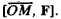# Vector Product

Also found in: Dictionary, Thesaurus, Wikipedia.
Related to Vector Product: vector multiplication

## vector product

[′vek·tər ‚präd·əkt]
(mathematics)

## Vector Product

The vector product of vector a and vector b is the vector denoted by [a, b] and defined as follows: (1) the length of vector [a, b] is equal to the product of the lengths of vectors a and b and the sine of the angle φ between them (the angle between a and b which is less than or equal to π is used); (2) vector [a, b] is perpendicular to both vectors a and b; and (3) the set of three vectors a, b, and [a, b], in accordance with its spatial orientation, always forms either a right-handed or left-handed set. The vector product is widely used in geometry, mechanics, and physics; for example, the moment of a force F, applied to a point M with reference to a point O, is the vector product### REFERENCE

Il’in, V. A., and E. G. Pozniak. Analiticheskaia geometriia. Moscow, 1968.

E. G. POZNIAK

References in periodicals archive ?
Matrix vector products are enhanced to O([N.sub.b][M.sub.b] log [N.sub.b][M.sub.b]) by performing a series of 1D discrete convolutions through the use of digital signal processing , with the x source and x observation coupling producing,
In Equation (6), the matrix vector product [Z.sub.EE] [I.sup.(k).sub.E] for interactions inside [[OMEGA].sub.E] is accelerated by the conventional MLFMA, in which the direct interaction between two far-field elements is separated into three steps containing aggregation, translation and disaggregation.
The spreading code of the code spur will be the vector product (assuming bipolar notation) of all the spreading codes in each permutation, and the code spur will have the same spreading factor as the codes used in each permutation.
* Vector Product Format Statistical (VPFStat) Software
We notice that each matrix vector product f(A)[u.sub.i], i = 1,2,...,m, can be approximately found by the one-term, two-term, and three-term vector estimates [[phi].sub.z] in (2.6), [[phi].sub.n,k] in (2.11), and [[phi].sub.n,k,l] in (2.19), respectively.
Because of the linearity of the vector product, the vector IP
Fix a vector product [pi] on V, and define I([delta],[epsilon]) = (V,[[xi].sub.[delta]],[epsilon][pi]) for ([delta],[epsilon]) [member of] P.
In , authors defined a vector product and by this way, they presented a method to calculate Frenet apparatus of space-like curves with non-null frame vectors and time-like curves in Minkowski space-time.
It is highly productive to start from the idea of the outer vector product known from mathematics: two vectors of identical size start in a coordinate origin and open up a plane.
The neutron spin ([s.sub.n]) is aligned to the direction of a vector product of the nuclear spin (I) and the neutron momentum ([k.sub.n]) for the measurement of a T-odd correlation term, which is represented as [s.sub.n] * ([k.sub.n] X I), during propagation through a polarized nuclear target.
It is extremely productive to give the resultant vector (EV) from the outer vector product (Part I of this article series) a physical significance.

Site: Follow: Share:
Open / Close